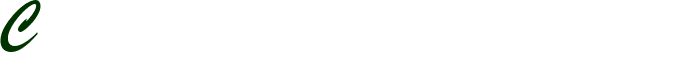# Individual Java 3

Individual Java 3

Field: Information Systems

Write a Java™ console application (not a Graphical User Interface application – you can use your Week 2 assignment as a starting point) using Java™ NetBeans™ IDE to meet these additional and changed business requirements:

The company has recently changed its total annual compensation policy to improve sales.

A salesperson will continue to earn a fixed salary of \$30,000.

The total annual compensation is the fixed salary plus the commission earned.

The current sales target for every salesperson is \$400,000.

Sales incentives are calculated as follows:

The sales incentive will only start when 80% of the sales target is met. The sales person will earn zero commission until 80% of the sales target is achieved.

The current commission is 8% of total sales.

If a salesperson exceeds the sales target, the commission rate will increase based on an acceleration factor. The acceleration factor is 1.25 (which means that the commission rate for salespersons that achieve the sales target is 10%, that is, 1.25 x 8%= 10%).

For clarification, the effective commission rates are as follows:

For total annual sales of \$0.00 to 319,999.99, the commission rate is 0% of total annual sales.

For total annual sales of \$320,000.00 to 399,999.99, the commission rate is 8% of total annual sales.

For total annual sales of \$400,000.00 and above, the commission rate is 10% of total annual sales.

The application should ask the user to enter annual sales, and it should display the total annual compensation.

The application should also display a table of potential total annual compensation that the salesperson could have earned, in \$5000 increments above the salesperson’s annual sales, until it reaches 50% above the salesperson’s annual sales.

Sample Table: Assuming a total annual sales of \$100,000, the table would look like this:

Total Sales    Total Compensation

\$100,000       <<Program calculated value, in this case \$30,000>>

\$105,000       <<Program calculated value, in this case \$30,000>>

\$110,000       <<Program calculated value, in this case \$30,000>>

\$115,000       <<Program calculated value, in this case \$30,000>>

\$120,000       <<Program calculated value, in this case \$30,000>>

\$125,000       <<Program calculated value, in this case \$30,000>>

\$130,000       <<Program calculated value, in this case \$30,000>>

\$135,000       <<Program calculated value, in this case \$30,000>>

\$140,000       <<Program calculated value, in this case \$30,000>>

\$145,000       <<Program calculated value, in this case \$30,000>>

\$150,000       <<Program calculated value, in this case \$30,000>>

The Java™ application should also meet these technical requirements:

The source code must demonstrate the use of looping structures.

The application should have at least one class, in addition to the application’s controlling class (a controlling class is where the main function resides). This means you must write and use at least two classes to meet the requirements. Simply using a second class that somebody else wrote does not meet the requirement (see the student material within the Week 2 Supporting Activity “Discussion of a Three Class Example” to understand how to write multiple classes).

There should be proper documentation in the source code including any references used (references used should be in a “References” section of comments at the end of your file).

Submit the “.java” file(s). Do NOT submit a .zip or .jar file or a compiled .class file, only submit the .java file(s). Do not submit a screen shot.

Note: Use common sense regarding the table. The “Program calculated value” in the <<>> markings above do not mean to type out the words “Program calculated value”, but to actually have a value print out in this location that is calculated based upon the corresponding annual sales value in the left column.

Tip: To make this table the easiest way is by using the tab escape sequence “\t” to separate the columns within a System.out.println statement which is called within a loop counting Total Sales using the +=5000 operator and by using a method to determine Total Compensation as a return value for the method that has Total Sales as an input value.## You Need a Professional Writer To Work On Your Paper?## Do you have an Urgent Assignment?;
BestQualityExperts

Disclaimer: The services that we offer are meant to help the buyer by providing a guideline.
The product provided is intended to be used for research or study purposes only.